# Displacement and Velocity INTRODUCTION TO MECHANICS DISTANCE VS

• Slides: 16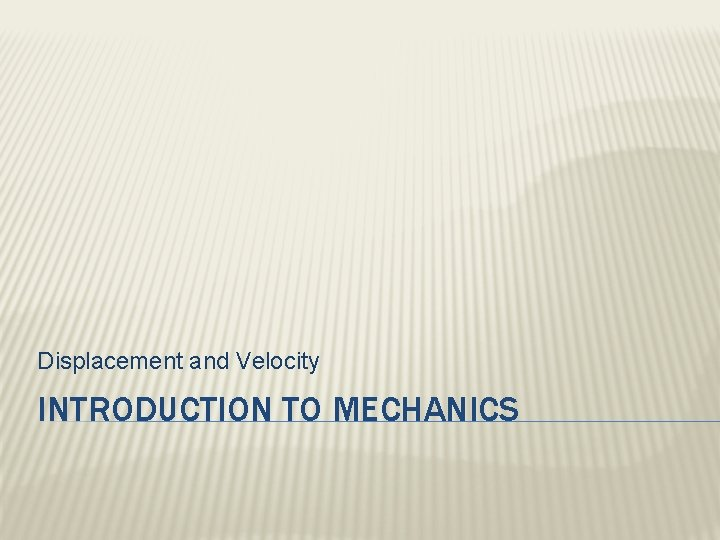Displacement and Velocity INTRODUCTION TO MECHANICSDISTANCE VS. DISPLACEMENT � Distance: the total length of the path taken to move from a starting point to an ending point, regardless of how many turns and changes of direction occur. (scalar quantity) � Displacement: The length of a straight line path between a starting point and an ending point. (vector quantity) � The change in position between an object’s initial position and final position.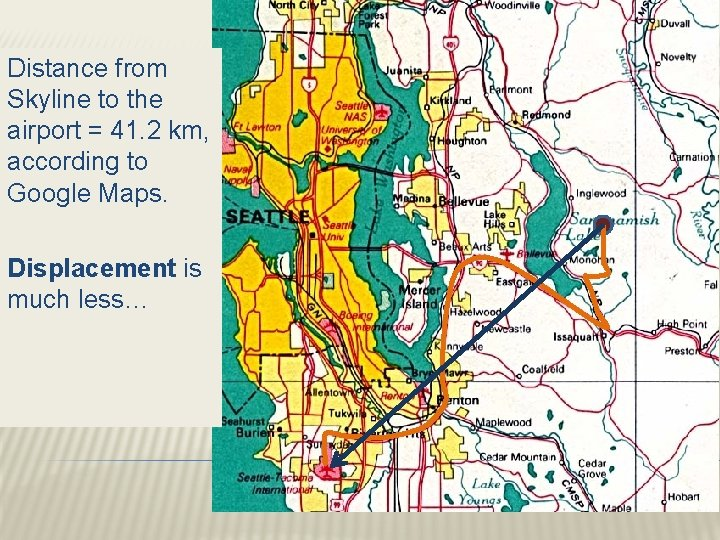Distance from Skyline to the airport = 41. 2 km, according to Google Maps. Displacement is much less…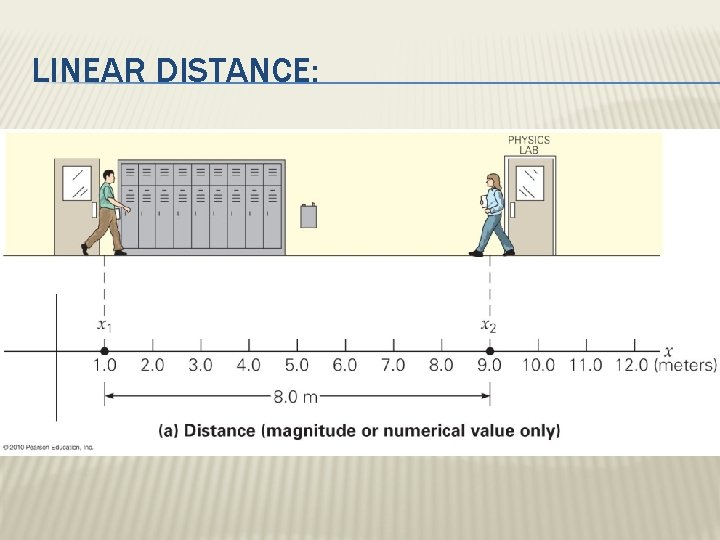LINEAR DISTANCE: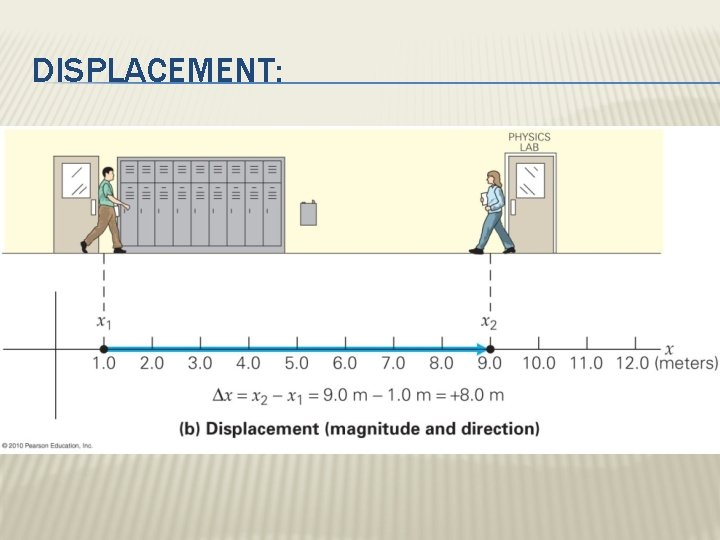DISPLACEMENT:SPEED VS. VELOCITY � Average Speed: the rate at which a distance is traveled: � In this case, d represents a TOTAL DISTANCE covered in a total time, t � Units for average speed = m∙s-1SPEED VS. VELOCITY � Average Velocity: the rate of a change in position. � In other words…the rate at which a DISPLACEMENT is traveled: �s represents DISPLACEMENT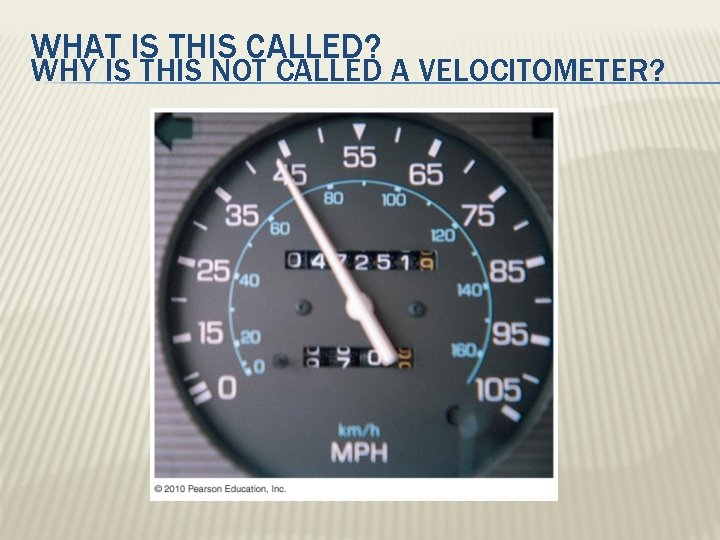WHAT IS THIS CALLED? WHY IS THIS NOT CALLED A VELOCITOMETER?SAMPLE PROBLEM: � While chasing an impala, a Cheetah runs 275 m at an average speed of 70. 0 mi/h Assuming that the impala is caught at the end of the 275 m, how much time elapses between when the cheetah begins its sprint and when he catches his prey?SAMPLE PROBLEM 2 �A right-handed baseball player hits a home run and takes 25. 0 s to jog around the bases. Assume that the total distance he runs is 115 m (he rounds the bases widely). � What is his average speed for his trip around the bases? � What, approximately, is his average velocity?LAB: PAPER RIVER � Form groups of 3 (2 is tricky, but doable) � Read through the procedure and make a plan for how you are going to proceed.INSTANTANEOUS VELOCITY � Average velocity will be used to describe the total displacement traveled in a total amount of time � Instantaneous velocity describes how fast an object is traveling at one given instant of time. � Examples: � The most common instantaneous velocities we will be using this year are: � Initial Velocity (vi or v 0 or u) � Final Velocity (vf or v)RELATIVE VELOCITY � The velocity of one object relative to another’s point of view � Reference Frame: the frame of reference for the observer’s point of view—the observer will always be at rest in his/her frame of reference � Relative velocity of an object moving relative to an observer is determined by subtracting vectors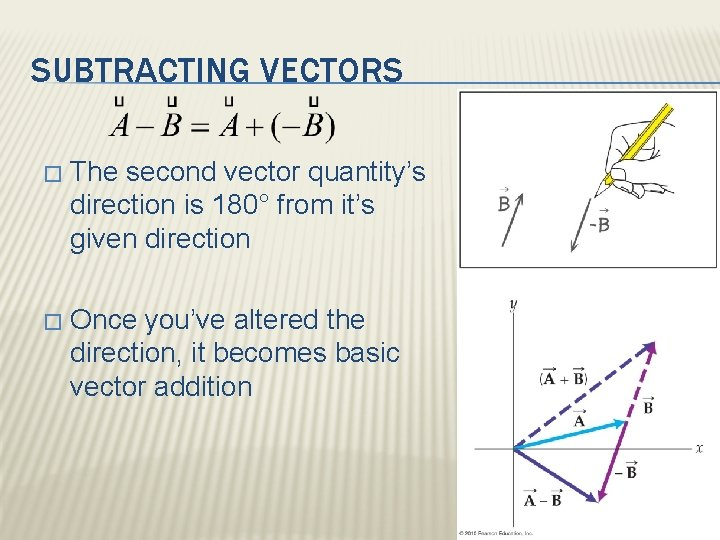SUBTRACTING VECTORS � The second vector quantity’s direction is 180° from it’s given direction � Once you’ve altered the direction, it becomes basic vector additionSAMPLE PROBLEM 1: � 1. 2. 3. 4. 5. 6. What is the velocity of: Car B relative to A? Car C relative to B? Car B relative to C? Car A relative to B?PRACTICE PROBLEM 2: � Car P moves to the left with a speed of 40. 0 km·h-1 (with respect to the road) and Car Z moves to the right with a speed of 60. 0 km·h-1 (with respect to the road). Find the velocity of car Z relative to car P. (Assume left is (-))